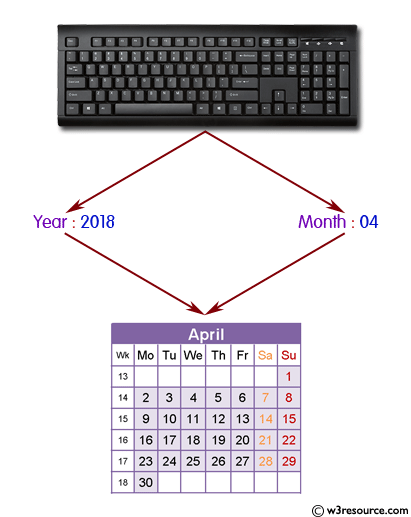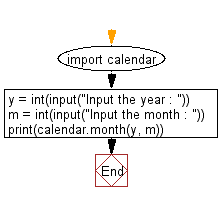﻿ Python: Print the calendar of a given month and year - w3resource# Python: Print the calendar of a given month and year

## Python Basic: Exercise-12 with Solution

Write a Python program to print the calendar of a given month and year.

Note: Use 'calendar' module.

Python calendar.month(theyear, themonth, w=0, l=0):

The function returns a month’s calendar in a multi-line string using the formatmonth() of the TextCalendar class.

'l' specifies the number of lines that each week will use.

Pictorial Presentation:Sample Solution:-

Python Code:

``````import calendar
y = int(input("Input the year : "))
m = int(input("Input the month : "))
print(calendar.month(y, m))```
```

Sample Output:

```Input the year : 2017
Input the month : 04
April 2017
Mo Tu We Th Fr Sa Su
1  2
3  4  5  6  7  8  9
10 11 12 13 14 15 16
17 18 19 20 21 22 23
24 25 26 27 28 29 30
```

Flowchart:Python Code Editor:

Have another way to solve this solution? Contribute your code (and comments) through Disqus.

What is the difficulty level of this exercise?

Test your Python skills with w3resource's quiz

﻿

## Python: Tips of the Day

List comprehension:

```>>> m = [x ** 2 for x in range(5)]
>>> m
[0, 1, 4, 9, 16]
```